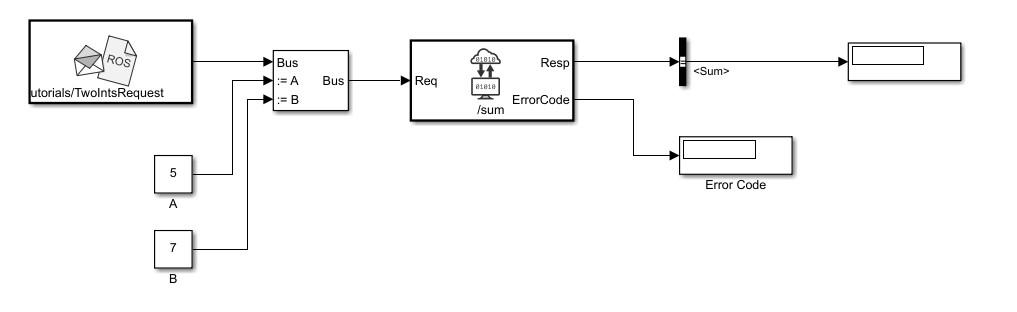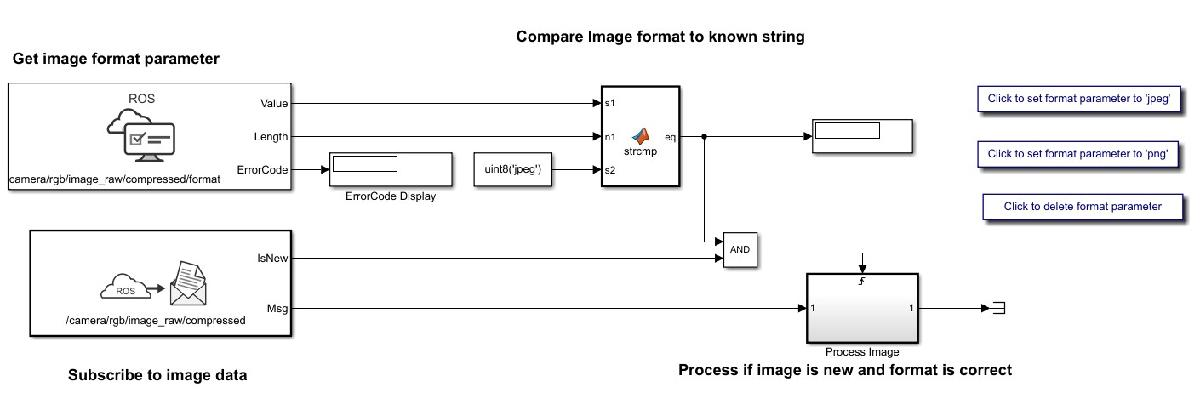These examples show how to get, set, compare, and manipulate ROS parameters in Simulink®. To run these examples, you must first set up a ROS network using `rosinit`. To set network-wide settings and share values with the whole network, start a ROS parameter server using `rosparam`. Follow these examples to see how to work with parameters in Simulink, including using string parameters.

### Get and Set ROS Parameters

This model gets and sets ROS parameters using Simulink®. This example illustrates how to use ROS parameters in Simulink and to share data over the ROS network. An integer value is set as a parameter on the ROS network. This integer is retrieved from the parameter server and compared to a constant. The output Boolean from the comparison is also set on the network. Change the constant block in the top left (blue) when you run the model to set network parameters based on user input conditions.

You must be connected to a ROS network. Call `rosinit` in the MATLAB® command line.### Set String Parameter on ROS Network

To create your string parameter, use a String Constant block and convert it to uint8 using a MATLAB function block. The converted uint8 string is passed into the Set Parameter block along with the extra input, Length, specified with a second Constant block. The Length refers to the maximum expected string length and is required for all string parameters. For more information, see the Set Parameter block.### Compare ROS String Parameters

On ROS networks, strings parameters are stored as a `uint8` array. When you get from string parameters from the server, they are returned as a `char` array. In Simulink®, they are cast as `uint8`, so you must use `uint8` character vectors when comparing to the ROS string parameters. You can use this comparison to trigger subsystems for larger models or validate settings for specific algorithms.

Connect to a ROS network. Set up the ROS Parameter tree.

`rosinit`
```Launching ROS Core... ...........................................Done in 2.0825 seconds. Initializing ROS master on http://192.168.0.10:60912. Initializing global node /matlab_global_node_60697 with NodeURI http://bat1012501glnxa64:41601/ ```
`ptree = rosparam;`

Set a ROS parameter, `/camera_format`, to a string value. You can use string scalars or character vectors. The value is stored as a `uint8` array on the ROS parameter server and returned as `'jpeg'` in MATLAB®.

```set(ptree,"/camera_format","jpeg") pause(1) pvalue = get(ptree,"/camera_format")```
```pvalue = 'jpeg' ```

Run the attached Simulink® model. This model checks to see if the previously set camera format parameter is named 'jpeg'. To get the parameter off the server, use the Get Parameter block. Then, compare the parameter to a character vector cast as `uint8` from a Constant block, using a MATLAB function block. An output of 1 means the parameters match.

```open_system("rosStringParameterCompare") sim("rosStringParameterCompare");```Shutdown ROS network.

`rosshutdown`
```Shutting down global node /matlab_global_node_60697 with NodeURI http://bat1012501glnxa64:41601/ Shutting down ROS master on http://192.168.0.10:60912. ........... ```

The `stringCompare` function is defined as:

```function y = stringCompare(str1,str2) %#codegen minLength = min(length(str1),length(str2)); st1 = str1(1:minLength); st2 = str2(1:minLength); y = all(st1(:)==st2(:)); ```

### Check Image Encoding Parameter for ROS Image Message

This model shows how to access string parameters and use them to trigger subsystem operations. It gets an image format off the set up ROS parameter server. It is retrieved as a `uint8` array that is compared using the `strcmp` MATLAB function block. When a new image is received from the Subscribe block and the format is `uint8('jpeg')`, it triggers the "Process Image" block to perform a task on the image data.

Connect to a ROS network and set up the ROS parameter server.

`rosinit`
```Launching ROS Core... ......................................Done in 1.3058 seconds. Initializing ROS master on http://192.168.0.10:55970. Initializing global node /matlab_global_node_28493 with NodeURI http://bat1012501glnxa64:44225/ ```
`ptree = rosparam;`

Set the `"/camera/rgb/image_raw/compressed/format"` parameter, and set up a publisher for the `"/camera/rgb/image_raw/compressed"` topic.

```set(ptree,"/camera/rgb/image_raw/compressed/format","jpeg") pub = rospublisher("/camera/rgb/image_raw/compressed","sensor_msgs/CompressedImage");```

Open the Simulink® model. This model checks the image format parameter and compares the value to a `uint8` cast character vector, `uint8('jpeg')` using a MATLAB® Function block. The boolean output is fed to an AND operator with the IsNew output of a Subscribe block that gets the image off the network. If the parameter value is correct and a new message is received, the Subsystem "Process Image" is triggered.

Run the model and use the buttons in the model to change the image format parameter and verify the `strcmp` function works. The `eq` output should be 1 when the parameter is set to `'jpeg'`. While the model is running, it is expected that image messages are being published on the network.

`open_system("rosImageFormatParameter")`Shut down the ROS network.

`rosshutdown`
```Shutting down global node /matlab_global_node_28493 with NodeURI http://bat1012501glnxa64:44225/ Shutting down ROS master on http://192.168.0.10:55970. ........ ```

The `strcmp` function in the MATLAB® Function block is defined as:

```function eq = strcmp(s1, n1, s2) %#codegen ```
```% Convert to proper strings string1 = char(s1(1:n1)); string2 = char(s2); ```
```eq = strcmp(string1, string2); ```